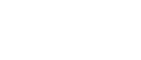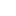#• PRODUCTS• SOLUTIONS• TUTORIALS• SUPPORTGlobal | English• Global
• United States
• Europe
• China
• Korea
• Japan

#• PRODUCTS
• SOLUTIONS
• TUTORIALS
• SUPPORT

# Current Sensor ICs

Low heat generation, fast response time, high accuracy,
high S/N Ratio in Open-type coreless current sensor ICs.

PRODUCTS

Asahi Kasei Microdevices (AKM), the Hall element leader, has developed advanced current sensors "Currentier" using the high-sensitivity compound semiconductor technology it has cultivated through its Hall elements, combined with Asahi Kasei Microdevices' advanced package design and analog circuit design technologies.

The name "Currentier" was made by combining "Current" and "Frontier". We named our product Currentier because it is a leading product in the field of current sensors thanks to its superb performance.

"Currentier" is magnetic open-type current sensor with a built-in high-sensitivity Hall element. They can detect both DC and AC currents quickly and with a high degree of accuracy. Despite their small size, they generate less heat and have high isolation. Currentiers are safe, high performance products that are available in many models and which can be used for current detection in a variety of applications, helping solve your problems.

## What makes AKM different ?

Features of Currentier

1. Low heat generation
2. Wide current detection range
3. High speed
4. High isolation
5. High resolution and high accuracy

## Solutions

Currentier can contribute to solving various problems of control equipment with the above features.

## Basic knowledge of current detection

Currentiers are classified as coreless current sensors, which is one of the current sensing methods. Other current detection methods include shunt resistor + insulated amplifier/insulated ADC and cored current sensors.

• Types and Characteristics of Current Sensors
• What is a coreless current sensor ？

Tutorials

## Search by Application

Currentiers can be used in a variety of applications.
The "Application" section contains examples of how Currentiers can be used to solve problems in individual applications, as well as recommendations regarding which products to use in each application.

• AC servo / General Inverter (Drives) for Industry
• Photovoltaic Inverter

Applications

## Search by Products

There is an extensive lineup of Currentier series that can be used for various applications.
In the "Lineup" section, you can select products based on their performance and specifications.

Product Map / Selection Guide

Lineup

## For Engineers under design or Users

In the "Design Support" section, you can find various tools, documents, FAQs, etc. for your design.

Datasheets / Application Notes / Tools / Circuit Examples / FAQ

Design Support

## Selection Table

`Part Number`
```Part
Status```
```Package Type
(Mounting style)```
```Maximum
Effective Current
[Arms]```
```Linear Sensing
Range (MIN.)
[A]```
```Linear Sensing
Range (MAX.)
[A]```
```Sensitivity
[mV/A]```
```Response Time
[μsec]```
```Power Supply
Voltage (MIN.)
[V]```
```Power Supply
Voltage (TYP.)
[V]```
```Power Supply
Voltage (MAX.)
[V]```
`Other`
```Operating
Temperature
[℃]```
```Device
Package```
```Number
of Pins```
`AK3101`
`EOL`
`SMD`
`20`
`-10.7`
`10.7`
`195`
`2`
`4.5`
`5.0`
`5.5`

`-40 to 90`
`SOP`
`14`
`AK3102`
`EOL`
`SMD`
`20`
`-21`
`21`
`100`
`2`
`4.5`
`5.0`
`5.5`

`-40 to 90`
`SOP`
`14`
`AK3103`
`EOL`
`SMD`
`20`
`-35`
`35`
`60`
`2`
`4.5`
`5.0`
`5.5`

`-40 to 90`
`SOP`
`14`
`AK310H`
`EOL`
`SMD`
`20`
`-42`
`42`
`50`
`2`
`4.5`
`5.0`
`5.5`

`-40 to 90`
`SOP`
`14`
`CQ2063`
`EOL`
`through hole type`
`50`
`-35`
`35`
`60`
`1`
`4.5`
`5.0`
`5.5`

`-40 to 90`
`SIP`
`7`
`CQ2064`
`EOL`
`through hole type`
`50`
`-54`
`54`
`40`
`1`
`4.5`
`5.0`
`5.5`

`-40 to 90`
`SIP`
`7`
`CQ2065`
`EOL`
`through hole type`
`50`
`-85`
`85`
`25`
`1`
`4.5`
`5.0`
`5.5`

`-40 to 90`
`SIP`
`7`
`CQ206A`
`EOL`
`through hole type`
`50`
`-46`
`46`
`45`
`1`
`4.5`
`5.0`
`5.5`

`-40 to 90`
`SIP`
`7`
`CQ206B`
`EOL`
`through hole type`
`50`
`0`
`45`
`90`
`1`
`4.5`
`5.0`
`5.5`

`-40 to 90`
`SIP`
`7`
`CQ2092`
`EOL`
`SMD`
`20`
`-21`
`21`
`100`
`1`
`4.5`
`5.0`
`5.5`

`-40 to 90`
`SIP`
`7`
`CQ2093`
`EOL`
`SMD`
`20`
`-35`
`35`
`60`
`1`
`4.5`
`5.0`
`5.5`

`-40 to 90`
`SIP`
`7`
`CQ209A`
`EOL`
`SMD`
`20`
`-31.8`
`31.8`
`66`
`1`
`4.5`
`5.0`
`5.5`

`-40 to 90`
`SIP`
`7`
`CQ209B`
`EOL`
`SMD`
`20`
`-35`
`0`
`115`
`1`
`4.5`
`5.0`
`5.5`

`-40 to 90`
`SIP`
`7`
`CQ209D`
`EOL`
`SMD`
`20`
`-41`
`41`
`50`
`1`
`4.5`
`5.0`
`5.5`

`-40 to 90`
`SIP`
`7`
`CQ2232`
`Preview`
`through hole type`
`50`
`-21`
`21`
`62.5`
`1`
`2.7`
`3.3`
`3.63`

`-40 to 110`
`SIP`
`7`
`CQ2233`
`Preview`
`through hole type`
`50`
`-35`
`35`
`37.5`
`1`
`2.7`
`3.3`
`3.63`

`-40 to 110`
`SIP`
`7`
`CQ2234`
`Preview`
`through hole type`
`50`
`-54`
`54`
`24.5`
`1`
`2.7`
`3.3`
`3.63`

`-40 to 110`
`SIP`
`7`
`CQ2235`
`Preview`
`through hole type`
`50`
`-85`
`85`
`15.5`
`1`
`2.7`
`3.3`
`3.63`

`-40 to 110`
`SIP`
`7`
`CQ2332`
`Preview`
`through hole type`
`50`
`-21`
`21`
`100`
`1`
`4.5`
`5.0`
`5.5`

`-40 to 110`
`SIP`
`7`
`CQ2333`
`Preview`
`through hole type`
`50`
`-35`
`35`
`60`
`1`
`4.5`
`5.0`
`5.5`

`-40 to 110`
`SIP`
`7`
`CQ2334`
`Preview`
`through hole type`
`50`
`-54`
`54`
`40`
`1`
`4.5`
`5.0`
`5.5`

`-40 to 110`
`SIP`
`7`
`CQ2335`
`Preview`
`through hole type`
`50`
`-85`
`85`
`25`
`1`
`4.5`
`5.0`
`5.5`

`-40 to 110`
`SIP`
`7`
`CQ2366`
`Preview`
`through hole type`
`50`
`-170`
`170`
`12`
`1.5`
`4.5`
`5.0`
`5.5`

`-40 to 110`
`SIP`
`7`
`CQ236B`
`EOL`
`through hole type`
`50`
`0`
`25`
`160`
`1`
`4.5`
`5.0`
`5.5`

`-40 to 110`
`SIP`
`7`
`CQ3200`
`Preview`
`SMD`
`20`
`-8.5`
`8.5`
`155`
`0.5`
`2.7`
`3.3`
`3.63`

`-40 to 90`
`VSOP`
`10`
`CQ3201`
`Preview`
`SMD`
`20`
`-10.1`
`10.1`
`130`
`0.5`
`2.7`
`3.3`
`3.63`

`-40 to 90`
`VSOP`
`10`
`CQ3202`
`Preview`
`SMD`
`20`
`-21.1`
`21.1`
`62.5`
`0.5`
`2.7`
`3.3`
`3.63`

`-40 to 90`
`VSOP`
`10`
`CQ3203`
`Preview`
`SMD`
`20`
`-35.7`
`35.7`
`37`
`0.5`
`2.7`
`3.3`
`3.63`

`-40 to 90`
`VSOP`
`10`
`CQ3204`
`Preview`
`SMD`
`20`
`-44`
`44`
`30`
`0.5`
`2.7`
`3.3`
`3.63`

`-40 to 90`
`VSOP`
`10`
`CQ320A`
`Preview`
`SMD`
`20`
`-29.3`
`29.3`
`45`
`0.5`
`2.7`
`3.3`
`3.63`

`-40 to 90`
`VSOP`
`10`
`CQ320B`
`Preview`
`SMD`
`20`
`-1.1`
`20.9`
`120`
`0.5`
`2.7`
`3.3`
`3.63`

`-40 to 90`
`VSOP`
`10`
`CQ3300`
`Preview`
`SMD`
`20`
`-6.4`
`6.4`
`325`
`0.5`
`4.5`
`5.0`
`5.5`

`-40 to 90`
`VSOP`
`10`
`CQ3301`
`Preview`
`SMD`
`20`
`-10.7`
`10.7`
`195`
`0.5`
`4.5`
`5.0`
`5.5`

`-40 to 90`
`VSOP`
`10`
`CQ3302`
`Preview`
`SMD`
`20`
`-21`
`21`
`100`
`0.5`
`4.5`
`5.0`
`5.5`

`-40 to 90`
`VSOP`
`10`
`CQ3303`
`Preview`
`SMD`
`20`
`-35`
`35`
`60`
`0.5`
`4.5`
`5.0`
`5.5`

`-40 to 90`
`VSOP`
`10`
`CQ330A`
`Preview`
`SMD`
`20`
`-31.8`
`31.8`
`66`
`0.5`
`4.5`
`5.0`
`5.5`

`-40 to 90`
`VSOP`
`10`
`CQ330B`
`Preview`
`SMD`
`20`
`0`
`21`
`195`
`0.5`
`4.5`
`5.0`
`5.5`

`-40 to 90`
`VSOP`
`10`
`CQ330E`
`Preview`
`SMD`
`20`
`0`
`20.5`
`200`
`0.5`
`4.5`
`5.0`
`5.5`

`-40 to 90`
`VSOP`
`10`
`CQ330F`
`Preview`
`SMD`
`20`
`-2`
`42`
`80`
`0.5`
`4.5`
`5.0`
`5.5`

`-40 to 90`
`VSOP`
`10`
`CQ330G`
`Preview`
`SMD`
`20`
`-4.5`
`4.5`
`450`
`0.5`
`4.5`
`5.0`
`5.5`

`-40 to 90`
`VSOP`
`10`
`CQ330H`
`Preview`
`SMD`
`20`
`-42`
`42`
`50`
`0.5`
`4.5`
`5.0`
`5.5`

`-40 to 90`
`VSOP`
`10`
`CQ330J`
`Preview`
`SMD`
`20`
`-2`
`42`
`82.7`
`0.5`
`4.5`
`5.0`
`5.5`

`-40 to 90`
`VSOP`
`10`
`CZ3700`
`Preview`
`SMD`
`60`
`-5.3`
`5.3`
`400`
`1`
`4.5`
`5.0`
`5.5`

`-40 to 105`
`SOP`
`10`
`CZ3701`
`Preview`
`SMD`
`60`
`-10.7`
`10.7`
`200`
`1`
`4.5`
`5.0`
`5.5`

`-40 to 105`
`SOP`
`10`
`CZ3702`
`Preview`
`SMD`
`60`
`-21.5`
`21.5`
`100`
`1`
`4.5`
`5.0`
`5.5`

`-40 to 105`
`SOP`
`10`
`CZ3703`
`Preview`
`SMD`
`60`
`-36`
`36`
`60`
`1`
`4.5`
`5.0`
`5.5`

`-40 to 105`
`SOP`
`10`
`CZ3704`
`Preview`
`SMD`
`60`
`-54`
`54`
`40`
`1`
`4.5`
`5.0`
`5.5`

`-40 to 105`
`SOP`
`10`
`CZ3705`
`Preview`
`SMD`
`60`
`-86.4`
`86.4`
`25`
`1`
`4.5`
`5.0`
`5.5`

`-40 to 105`
`SOP`
`10`
`CZ3706`
`Preview`
`SMD`
`60`
`-180`
`180`
`12`
`1`
`4.5`
`5.0`
`5.5`

`-40 to 105`
`SOP`
`10`
`CZ3720`
`Preview`
`SMD`
`60`
`-0.4`
`10.3`
`400`
`1`
`4.5`
`5.0`
`5.5`

`-40 to 105`
`SOP`
`10`
`CZ3721`
`Preview`
`SMD`
`60`
`-0.8`
`20.6`
`200`
`1`
`4.5`
`5.0`
`5.5`

`-40 to 105`
`SOP`
`10`
`CZ3722`
`Preview`
`SMD`
`60`
`-1.7`
`41.3`
`100`
`1`
`4.5`
`5.0`
`5.5`

`-40 to 105`
`SOP`
`10`
`CZ3723`
`Preview`
`SMD`
`60`
`-2.9`
`68.8`
`60`
`1`
`4.5`
`5.0`
`5.5`

`-40 to 105`
`SOP`
`10`
`CZ3724`
`Preview`
`SMD`
`60`
`-4.4`
`103.4`
`40`
`1`
`4.5`
`5.0`
`5.5`

`-40 to 105`
`SOP`
`10`
`CZ3725`
`Preview`
`SMD`
`60`
`-7.1`
`165.8`
`25`
`1`
`4.5`
`5.0`
`5.5`

`-40 to 105`
`SOP`
`10`
`CZ3726`
`Preview`
`SMD`
`60`
`-14.9`
`345.2`
`12`
`1`
`4.5`
`5.0`
`5.5`

`-40 to 105`
`SOP`
`10`
`CZ3813`
`EOL`
`SMD`
`40`
`-33`
`33`
`60`
`2`
`4.5`
`5.0`
`5.5`

`-40 to 125`
`SOP`
`10`
`CZ3814`
`EOL`
`SMD`
`40`
`-50`
`50`
`40`
`2`
`4.5`
`5.0`
`5.5`

`-40 to 125`
`SOP`
`10`
`CZ3815`
`EOL`
`SMD`
`40`
`-60`
`60`
`33.3`
`2`
`4.5`
`5.0`
`5.5`

`-40 to 125`
`SOP`
`10`
`CZ3A01`
`Preview`
`SMD`
`50`
`-12.9`
`12.9`
`120`
`1`
`3.0`
`3.3`
`3.6`

`-40 to 85`
`SOP`
`10`
`CZ3A02`
`Preview`
`SMD`
`50`
`-15.5`
`15.5`
`100`
`1`
`3.0`
`3.3`
`3.6`

`-40 to 85`
`SOP`
`10`
`CZ3A03`
`Preview`
`SMD`
`50`
`-22.1`
`22.1`
`70`
`1`
`3.0`
`3.3`
`3.6`

`-40 to 85`
`SOP`
`10`
`CZ3A04`
`Preview`
`SMD`
`50`
`-32.2`
`32.2`
`48`
`1`
`3.0`
`3.3`
`3.6`

`-40 to 85`
`SOP`
`10`
`CZ3A05`
`Preview`
`SMD`
`50`
`-64.5`
`64.5`
`24`
`1`
`3.0`
`3.3`
`3.6`

`-40 to 85`
`SOP`
`10`
`CZ3A06`
`Preview`
`SMD`
`50`
`-103.3`
`103.3`
`15`
`1`
`3.0`
`3.3`
`3.6`

`-40 to 85`
`SOP`
`10`
`CZ3A07`
`Preview`
`SMD`
`50`
`-129.1`
`129.1`
`12`
`1`
`3.0`
`3.3`
`3.6`

`-40 to 85`
`SOP`
`10`
`CZ3AG1`
`Preview`
`SMD`
`50`
`-11.6`
`11.6`
`120`
`3.6`
`4.5`
`5`
`5.5`

`-40 to 85`
`SOP`
`10`
`CZ3AG2`
`Preview`

`50`
`-14`
`14`
`100`
`3.6`
`4.5`
`5.0`
`5.5`

`-40 to 85`
`SOP`
`10`
`CZ3AG3`
`Preview`

`50`
`-20.0`
`20.0`
`70`
`3.6`
`4.5`
`5.0`
`5.5`

`-40 to 85`
`SOP`
`10`
`CZ3AG4`
`Preview`
`SMD`
`50`
`-29.1`
`29.1`
`48`
`3.6`
`4.5`
`5`
`5.5`

`-40 to 85`
`SOP`
`10`
`CZ3AG5`
`Preview`
`SMD`
`50`
`-58.3`
`58.3`
`24`
`3.6`
`4.5`
`5`
`5.5`

`-40 to 85`
`SOP`
`10`
`CZ3AG6`
`Preview`
`SMD`
`50`
`-93.3`
`93.3`
`15`
`3.6`
`4.5`
`5`
`5.5`

`-40 to 85`
`SOP`
`10`
`CZ3AG7`
`Preview`

`50`
`-116.6`
`116.6`
`12`
`3.6`
`4.5`
`5.0`
`5.5`

`-40 to 85`
`SOP`
`10`